Study Materials

# NCERT Solutions for Class 8th Mathematics

Page 1 of 2

## Chapter 1. Rational Numbers

### Exercise 1.1

Exercise 1.1

Q1. Using appropriate properties find;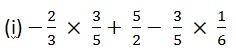Solution:Using BODMAS first we solve for multiply here;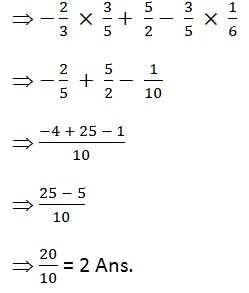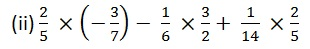Solution:Using BODMAS first we solve for multiply here;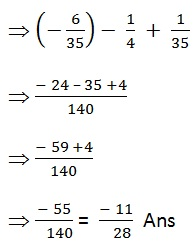Q2. Write the additive inverse of each of the following.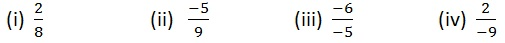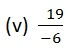Solution:Q3. Verify that – (- x) = x for;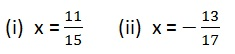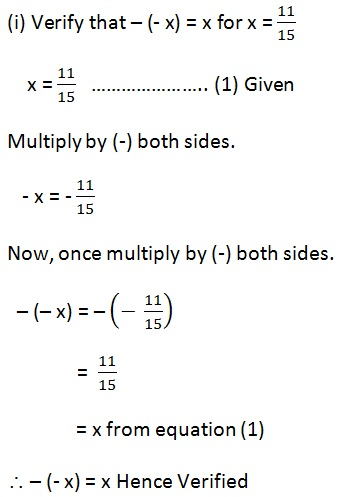Page 1 of 2

Chapter Contents: